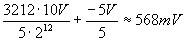Archived: Convert Traditional NI-DAQ (Legacy) Binary Data to Voltage Values

NI does not actively maintain this document.

This content provides support for older products and technology, so you may notice outdated links or obsolete information about operating systems or other relevant products.

Visão geral

Note: This page is about NI-DAQ also known as Traditional NI-DAQ (Legacy). NI-DAQmx replaced Traditional NI-DAQ (Legacy) in 2003. NI strongly recommends using NI-DAQmx for new designs and migrating existing Traditional NI-DAQ (Legacy) applications to NI-DAQmx. Please review the Getting Started with NI-DAQmx guide for more information on migration. Many Traditional NI-DAQ (Legacy) data acquisition functions return the actual binary codes returned by the A/D converter. This document explains how to convert the binary codes into voltage values.

Overview

Many Traditional  NI-DAQ (Legacy) data acquisition functions -- such as AI_Read, DAQ_Start, and SCAN_Op -- return binary codes. The LabVIEW AI Read.vi (in output type 2) also returns binary information. Generally, a call to AI_VScale returns the voltage value corresponding with a binary code, but you may want to manually calculate the value. For example, suppose you're acquiring data in one program and saving it to a file. Then, you have a second program that has no other DAQ functionality than to read the data in the file. The second program does not have to load nidaq32.dll in order to call AI_VScale. Instead, you can have the program do a simple mathematical calculation to convert the binary codes into voltage values.

The Information You Need

In order to convert from the binary code, you need the following information about the specific DAQ operation that acquired the data:

• The resolution of the board
• The gain
• The range
• The polarity

The codes, or individual binary values, are evenly distributed over the entire range of the A/D converter. The number of codes is dependent on the resolution of the board: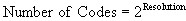Most of the National Instruments DAQ boards have 12-bit resolution, which means the boards have 4096 codes.

The range of the A/D converter for the National Instruments DAQ products is either -5 to +5 V, or 0 to 10 V, depending on the polarity setting. Notice that each range is 10 V in size. So, each of the 4096 codes is distributed over a 10 V range in either case.

However, you still need to factor in the pre-conversion gain, which effectively changes the range over which the codes are distributed. If, for example, a gain of 10 is applied, the effective range of the A/D converter is -0.5 to +0.5 V, and the codes are distributed over a 1 V range. Thus, each code is worth 1/10th as much voltage, resulting in higher accuracy.

Conversion Formulas

In order to calculate the voltage represented by a specific code, you need to know the code width, or the amount of voltage that each code represents. You calculate the code width using the following formula: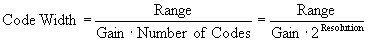For a typical E Series board with a resolution of 12 bits, and a range of -5 to +5 V set at a gain of 1, the code width is 2.4 mV.

By multiplying the code returned from the DAQ operation by the code width, you can calculate how far in volts the code was from the bottom of the range. All that's left is to remember what the gain adjusted range bottom was, and subtract that from the voltage offset: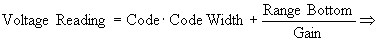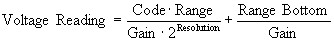For example, suppose a typical 12-bit E Series board returned a code of 3212 in bipolar mode (range = –5 to + 5 volts) with a gain of 5. The voltage value that corresponds is: